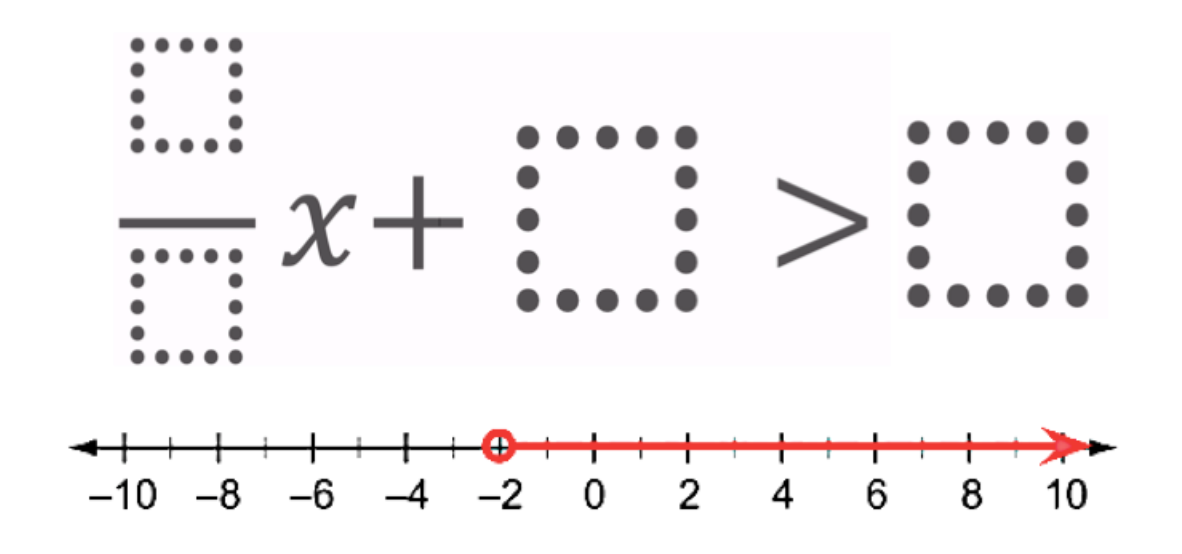# Two Step Inequality with Fractional Coeffcient

Directions: Using the digits 1 to 7 at most one time each, place a digit in each box to create an inequality that match the solution of the inequality on the number line.### Hint

What might be a way to get a negative number on the right side of the inequality?

(2/1)x + 7 > 3
(4/2)x + 7 > 3
(1/2)x + 3 > 4
(1/2)x + 5 > 6
(1/2)x + 6 > 7
(2/4)x + 5 > 6
(2/4)x + 6 > 7
(3/2)x + 4 > 1
(3/2)x + 7 > 4
(5/2)x + 6 > 1
(6/2)x + 7 > 1
(3/1)x + 7 > 1

Source: Jay Sydow

## Similar Triangles And Slope

Directions: The three triangles on the line are similar. Using the digits 0 to 9 …

1.Hello! I have a question about some of the answers that were listed. Please let me know if my thinking is correct.

I figured that the constant had to be larger than the “answer” since the graph shows that the solution is x > -2.

I wonder why answers like (1/2)x + 3 > 4, (1/2)x + 6 > 7 work as you get x >2, not x > -2

•(1/2)x + 3 > 4 should be (1/2)x + 4 > 3
(1/2)x + 5 > 6 should be (1/2)x + 6 > 5
(1/2)x + 6 > 7 should be (1/2)x + 7 > 6
(2/4)x + 5 > 6 should be (1/2)x + 6 > 5
(2/4)x + 6 > 7 should be (1/2)x + 3 > 4

Sorry about that. Thank you for checking that.